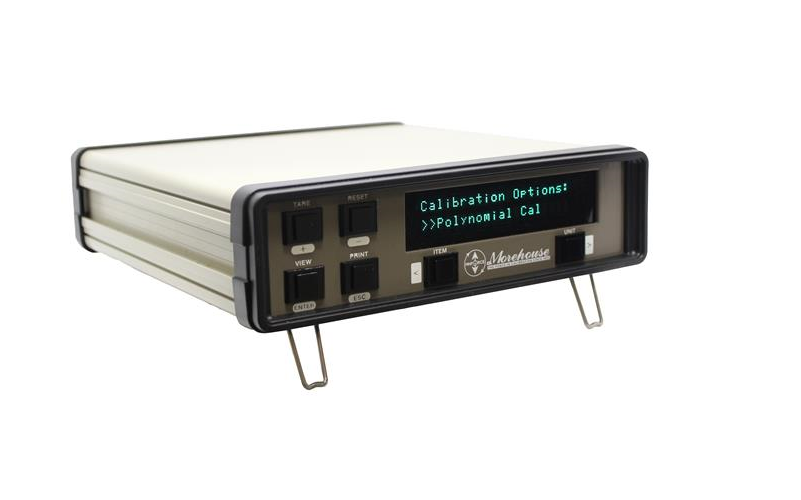# New 4215 Plus Indicator Lowers Measurement Error for Force Calibrations

The 4215 Plus indicator can store coefficients used to predict load cell deflection values throughout the calibrated range.Morehouse Instrument Company, Inc. (York, PA) has announced a new 4215 Plus indicator that uses a polynomial equation to reduce non-linear load cell errors. Many other indicators use a span calibration, which introduces significant bias (measurement error) because the load cell output often fits better to a calibration curve. Sometimes the bias from a calibration span is larger than the load cell specification. The bias adds more error and increases the measurement uncertainty.

The 4215 Plus indicator can store coefficients used to predict load cell deflection values throughout the calibrated range. The polynomial equation, fitted to calibration data using the least-squares method, is found on many ASTM E74, ISO 376, and other calibration certificates.

Calibrations are performed in mV/V and converted via the polynomial equation to various force units such as gf, lbf, kgf, kN, N and t. Any time the unit is sent in for calibration, a new polynomial equation is calculated and entered directly into the indicator. This means additional adjustments are not needed, which reduces calibration costs.

www.mhforce.com, 717-843-0081

Latest in New Products